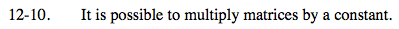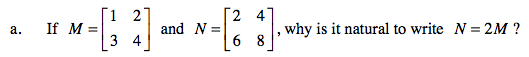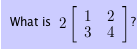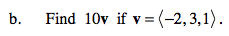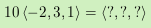### Home > PC > Chapter 12 > Lesson 12.1.1 > Problem12-10

12-10.
1. It is possible to multiply matrices by a constant. Homework Help ✎

1. If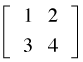and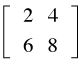, why is it natural to write N = 2M?

2. Find 10v if v =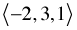.

When vectors are in component form, matrix operations can be performed. With the addition of matrices, many problems with vectors can be solved. The same rules apply when multiplying a vector by a matrix as multiplying two matrices together.